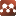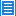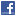## Classical Physics## Unification of Electromagnetism, Weak Gravitation, and Classical and Quantum Mechanics by Diamond Equations

Authors: Hideki Mutoh

It was found that electromagnetic and weak gravitational fields can be unified by diamond equations, which is one of extended Maxwell’s equations. Classical mechanics is derived from the diamond equation for weak gravitational field by assuming that gravitational wave is not generated under the condition of stable motion. Quantum mechanics is derived from the same equation by assuming that the square of total energy consists of square of total momentum, square of the mass, and imaginary part of the energy creation-annihilation rate.